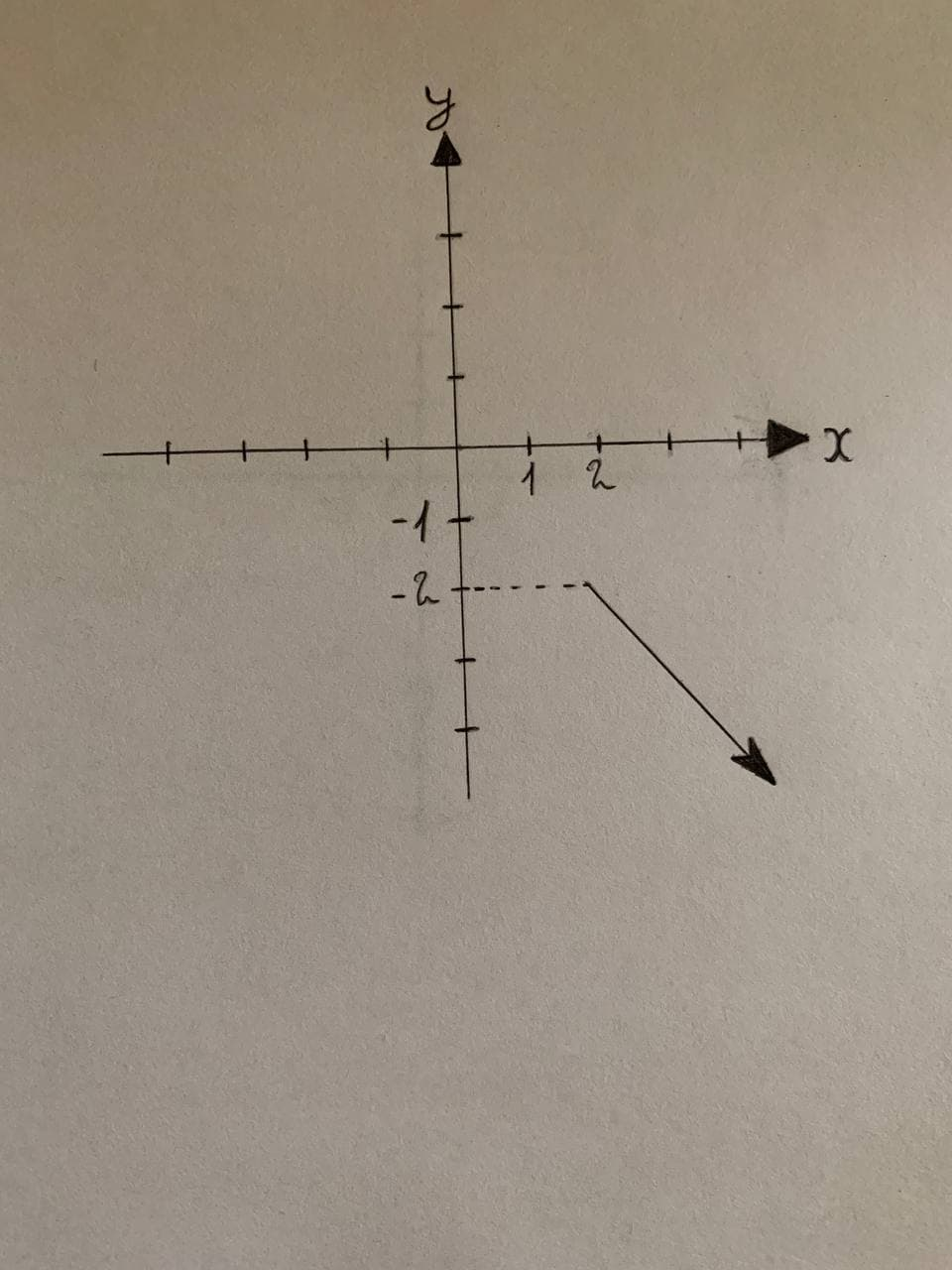Graph the sets of points whose polar coordinates satisfy theVirginia Caron 2021-11-22 Answered
Graph the sets of points whose polar coordinates satisfy the given polar equation.
The given polar equation is written as follows:
$$\displaystyle\theta={\frac{{{2}\pi}}{{{3}}}},{r}\le-{2}$$

• Questions are typically answered in as fast as 30 minutes

Solve your problem for the price of one coffee

• Math expert for every subject
• Pay only if we can solve itpendukke
Description:
Consider the polar coordinates as $$\displaystyle{P}{\left({r}.\theta\right)}$$.
Here, r is the directed distance from origin Oto point Pand $$\displaystyle\theta$$ is the directed angle from initial ray to OP.
From the given equation, the range of r is from —2 to $$\displaystyle—\propto$$ and 0s fixed at $$\displaystyle{\frac{{{2}\pi}}{{{3}}}}$$. Therefore, the polar point traces a straight line at an angle of $$\displaystyle{\frac{{{2}\pi}}{{{3}}}}$$ from the initial ray (x-axis) and the line also satisfies the range of r.
From the analysis, draw the sets of points whose polar coordinates satisfy the given polar equation as shown in Figure 1.
$$\displaystyle\theta={\frac{{{2}\pi}}{{{3}}}},$$
$$\displaystyle{r}\le-{2}$$